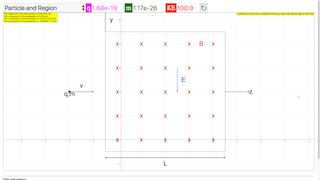Intro Page

The Wien (E x B) Filter

Developed by E. Behringer

This set of exercises guides the students to compute and analyze the behavior of a charged particle in a spatial region with mutually perpendicular electric and magnetic fields. It requires the student to determine the Cartesian components of hte forces acting on the particle and to obtain the corresponding equations of motion. The solutions to these equations are obtained through numerical integation, and the capstone exercise is the simulation of the $\stackrel{\to }{E}×\stackrel{\to }{B}$ (Wien) filter.

 Subject Area Electricity & Magnetism First Year and Beyond the First Year Python Students who complete this set of exercises will be able to: generate equations predicting the Cartesian components of force acting on the charged particle and generate the equations of motion for the particle (Exercise 1); calculate particle trajectories by solving the equations of motion (Exercise 2); produce two-dimensional and three-dimensional plots of the trajectories (Exercise 2); and simulate the operation of an E⃗ ×B⃗ $\stackrel{\to }{E}×\stackrel{\to }{B}$ (Wien) filter (Exercise 3) and determine the range of particle velocities transmitted by the filter, and how these are affected by the geometry of the filter (Exercise 4). 120 min
Exercise 1

### EXERCISE 1: FORCES ACTING ON A CHARGED PARTICLE AND THE EQUATIONS OF MOTION

Imagine that we have a particle of mass $m$, charge $q$, and velocity $\stackrel{\to }{v}=\left({v}_{x},{v}_{y},{v}_{z}\right)$ with ${v}_{z}>>{v}_{x},{v}_{y}$entering a region of uniform magnetic field $\stackrel{\to }{B}={B}_{x}\stackrel{^}{x}$ and uniform electric field $\stackrel{\to }{E}={E}_{y}\stackrel{^}{y}$. As shown below, ${B}_{x}>0$ and ${E}_{y}<0$. The length of the field region along the $z$-axis is $L$.

Neglecting any other forces (e.g., gravitational forces), show that the Cartesian components of the combined electric and magnetic forces are

$\begin{array}{}\text{(7)}& {F}_{x}=0\end{array}$
$\begin{array}{}\text{(8)}& {F}_{y}=q{E}_{y}+q{v}_{z}{B}_{x}\end{array}$
$\begin{array}{}\text{(9)}& {F}_{z}=-q{v}_{y}{B}_{x}\end{array}$

resulting in the equations of motion

$\begin{array}{}\text{(10)}& \stackrel{¨}{x}=0\end{array}$
$\begin{array}{}\text{(11)}& \stackrel{¨}{y}=\frac{q}{m}\left({E}_{y}+{v}_{z}{B}_{x}\right)\end{array}$
$\begin{array}{}\text{(12)}& \stackrel{¨}{z}=-\frac{q}{m}{v}_{y}{B}_{x}\end{array}$

where the dot accents indicate differentiation with respect to time. Note that the particle will not experience any transverse acceleration if ${v}_{z}={v}_{pass}=-{E}_{y}/{B}_{x}$.

(a) Assume that ${E}_{y}=-105$ V/m and ${B}_{x}=2.00×{10}^{-3}$ T, and that all other field components are zero. Calculate, by hand, the Cartesian components of the acceleration at the instant when a Li${}^{+}$ ion of mass 7 amu and kinetic energy 100 eV enters the field region traveling along the direction $\stackrel{^}{u}=\left(\stackrel{^}{x}+\stackrel{^}{y}+100\stackrel{^}{z}\right)/\sqrt{10002}$.

How will these acceleration components compare to those for a doubly ionized nitrogen ion (N${}^{+}$)?

(b) Write a code to perform the calculation in part (a). Note that, as soon as the particle enters the field region, the velocity components will change, and therefore so will the forces. To calculate an accurate trajectory, it is necessary to repeatedly calculate the forces, a task for which the computer is very well suited.

(c) What do you expect the trajectory of this ion to look like as it traverses the field region? Explain your answer.

ExB_Filter_Exercise_1.py with Bug Fix

#

# ExB_Filter_Exercise_1.py

#

# This file is used to calculate

# the Cartesian components of the acceleration of

# a charged particle through

# an E x B velocity filter.

#

# Here, it is assumed that the axis of the filter

# is aligned with the z-axis, that the magnetic field

# is along the +x-direction, and that the electric field

# is along the -y-direction.

#

# By:

# Ernest R. Behringer

# Department of Physics and Astronomy

# Eastern Michigan University

# Ypsilanti, MI 48197

# (734) 487-8799 (Office)

# This email address is being protected from spambots. You need JavaScript enabled to view it.

#

# Last updated:

#

# 20110309 = March 9, 2011 ERB Initial writing in Matlab.

# 20160616 ERB conversion from Matlab.

#

from numpy import sqrt

import time

#

# Initialize parameter values

#

q = 1.60e-19 # particle charge [C]

m = 7.0*1.67e-27 # particle mass [kg]

KE_eV = 100.0 # particle kinetic energy [eV]

Ex = 0.0 # Ex = electric field in the +x direction [N/C]

Ey = -105.0 # Ey = electric field in the +y direction [N/C]

Ez = 0.0 # Ez = electric field in the +z direction [N/C]

Bx = 0.002 # Bx = magnetic field in the +x direction [T]

By = 0.0 # By = magnetic field in the +x direction [T]

Bz = 0.0 # Bz = magnetic field in the +x direction [T]

D = 2.0 # D = diameter of the exit aperture [mm]

L = 0.25 # L = length of the crossed field region [mm]

u = [1.0,1.0,100.0]/sqrt(10002.0) # direction of the velocity vector

# Derived quantities

qoverm = q/m # charge to mass ratio [C/kg]

KE = KE_eV*1.602e-19 # particle kinetic energy [J]

vmag = sqrt(2.0*KE/m) # particle velocity magnitude [m/s]

v1x = vmag*u # v1x = x-component of the initial velocity [m/s]

v1y = vmag*u # v1y = y-component of the initial velocity [m/s]

v1z = vmag*u # v1z = z-component of the initial velocity [m/s]

#

# Calculate the Cartesian components of the acceleration

#

a_x = qoverm*(Ex + v1y*Bz - v1z*By)

a_y = qoverm*(Ey + v1z*Bx - v1x*Bz)

a_z = qoverm*(Ez + v1x*By - v1y*Bx)

print ("The magnitude of the initial velocity is %.3e"%vmag," m/s.") ##Frem:Added brackets

print ("The x-component of the acceleration is %.3e"%a_x," m/s$$^2$$.") #Frem:Added brackets

print ("The y-component of the acceleration is %.3e"%a_y," m/s$$^2$$.") #Frem:Added brackets

print ("The z-component of the acceleration is %.3e"%a_z," m/s$$^2$$.") #Frem:Added brackets

time.sleep(10)##Frem:Added Time to prevent the program from closing too fast

### Translations

Code Language Translator Run### Software Requirements

SoftwareRequirements

 Android iOS Windows MacOS with best with Chrome Chrome Chrome Chrome support full-screen? Yes. Chrome/Opera No. Firefox/ Samsung Internet Not yet Yes Yes cannot work on some mobile browser that don't understand JavaScript such as..... cannot work on Internet Explorer 9 and below

### CreditsFremont Teng; Loo Kang Wee; based on codes by E. Behringer

[text]

[text]

Research

[text]

[text]

[text]